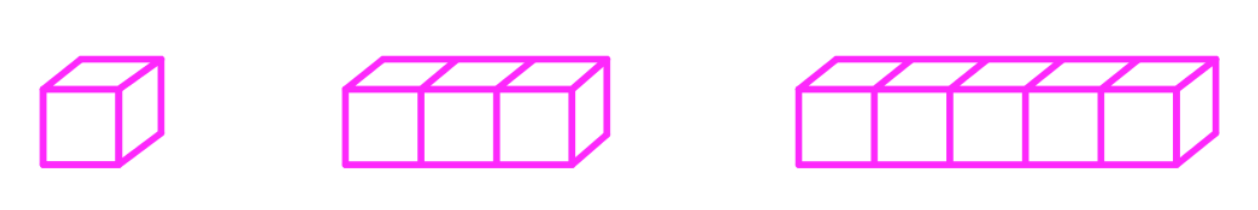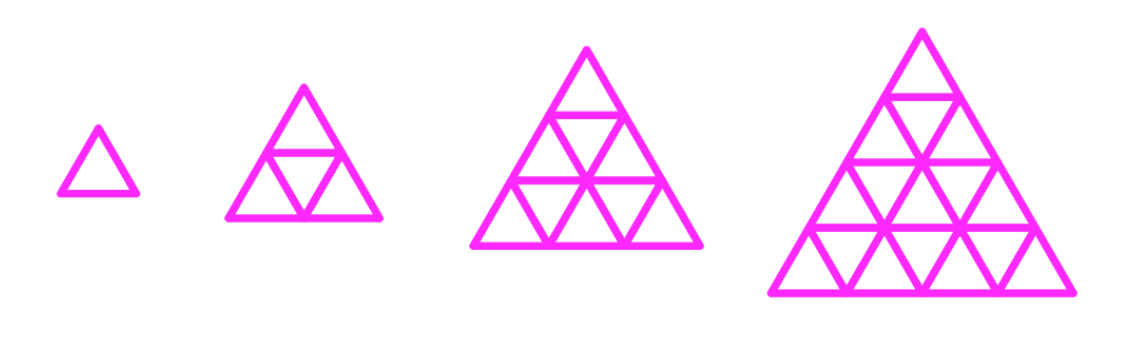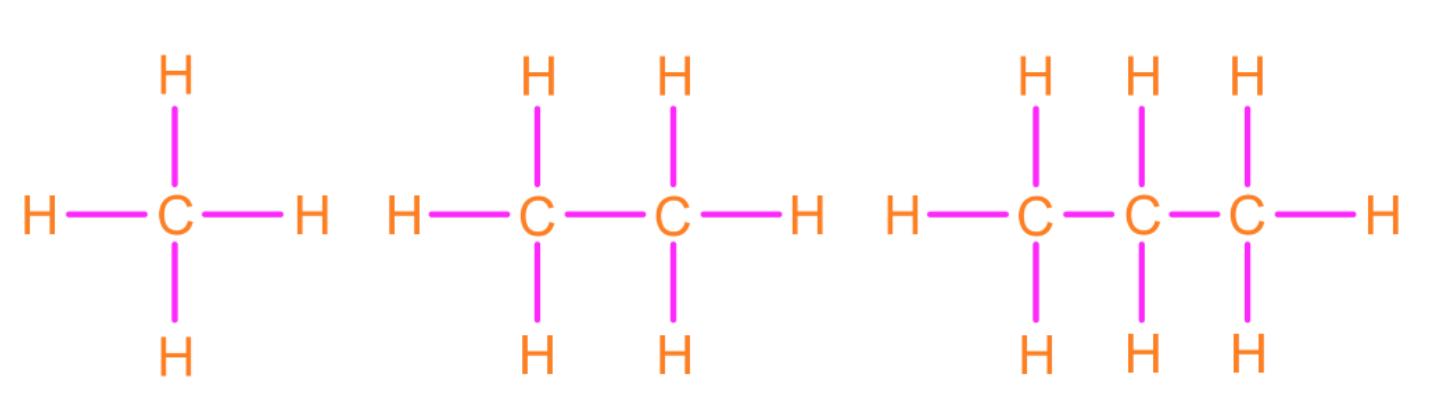# Inductive reasoning

### Inductive reasoning

#### Lessons

Notes:

A conjecture is an educational guess made from the given information. Inductive reasoning is about making a conjecture that predicts the next set of patterns, or arrive at a conclusion.
• Introduction
Inductive Reasoning Overview:
a)
What are conjectures?

• 1.
Making a Conjecture

Make a conjecture of the next item or number based on the information given:

a)
1, 3, 5, 7, 9,…

b)
$\frac{1}{2}, \frac{1}{3}, \frac{1}{4}, \frac{1}{5}$

c)d)e)f)• 2.
Make a conjecture with the given information. Draw a figure to show that your conjecture is correct:
a)
ABC is a triangle and AB = BC

b)
ABC is a triangle and $\angle$B is a right angle.

c)
Line $a$ and line $b$ are perpendicular

d)
Line $a$ and line $b$ are parallel

• 3.
Counterexamples of Conjectures
Determine if the following conjecture is true or false. If it is false, then find a counterexample of the conjecture:
a)
Given: A = (0,0), B = (0,1), C = (1,0).
Conjecture: ABC form a right isosceles triangle.

b)
Given: $a$ is a negative integer.
Conjecture: $\ a^2$ is a positive integer.

c)
Given: $\overline{AB}$ and $\overline{BC}$ are parallel
Conjecture: AB = BC

d)
Given: $x + y \geq 10 . x \geq 5$
Conjecture: $y \geq 6$